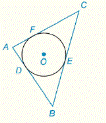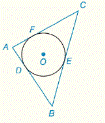Chapter 6.CR, Problem 34CR### Elementary Geometry for College St...

6th Edition
Daniel C. Alexander + 1 other
ISBN: 9781285195698

#### Solutions

Chapter
Section### Elementary Geometry for College St...

6th Edition
Daniel C. Alexander + 1 other
ISBN: 9781285195698
Textbook Problem
4 views

# G i v e n :                   ⊙ O   i s   i n s c r i b e d   i n   ∆ A B C                                                             A B = 9 ,   B C = 13 ,   A C = 10       F i n d :                           A D ,   B E ,   F CTo determine

To Find: AD, BE, FC if O is inscribed in ABC and AB=9, BC=13, AC=10.Explanation

Concept:

Theorem 6.3.4:

The tangent segments to a circle from an external point are congruent.

Calculation:

Given that O is inscribed in ABC and AB=9, BC=13, AC=10.

Let A,B, and C be vertices of the triangle.

AB,BC, and CA are the sides of the triangle.

Also, AB,BC,  and CA are the tangents of the circle at D, E and F respectively.

By Theorem 6.3.4:

The tangent segments to a circle from an external point are congruent.

Because AB=9, BC=13, AC=10

y+z=9

x+z=13

x+y=10

ABC is right triangle,

### Still sussing out bartleby?

Check out a sample textbook solution.

See a sample solution

#### The Solution to Your Study Problems

Bartleby provides explanations to thousands of textbook problems written by our experts, many with advanced degrees!

Get Started

#### CHECK POINT 2. (a) W rite as radicals. (b)Write with fractional exponents:

Mathematical Applications for the Management, Life, and Social Sciences

#### Sometimes, Always, or Never: If 0 ≤ an ≤ bn for all n and diverges, then converges.

Study Guide for Stewart's Single Variable Calculus: Early Transcendentals, 8th

#### Simplify each expression. 3i5

College Algebra (MindTap Course List)